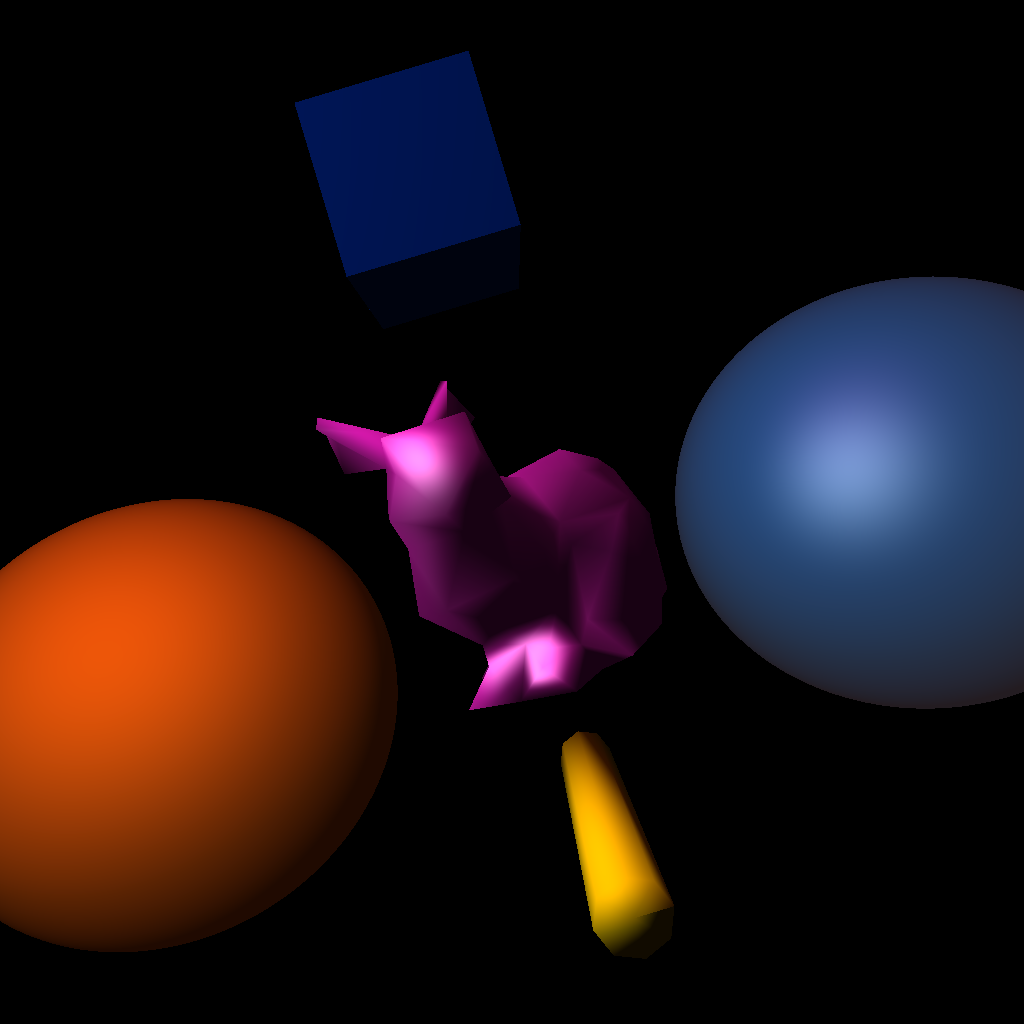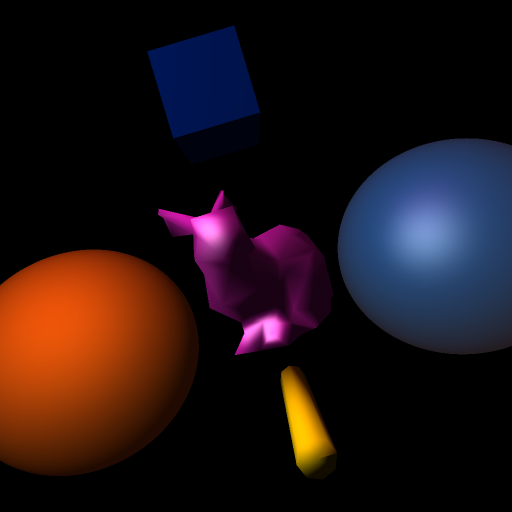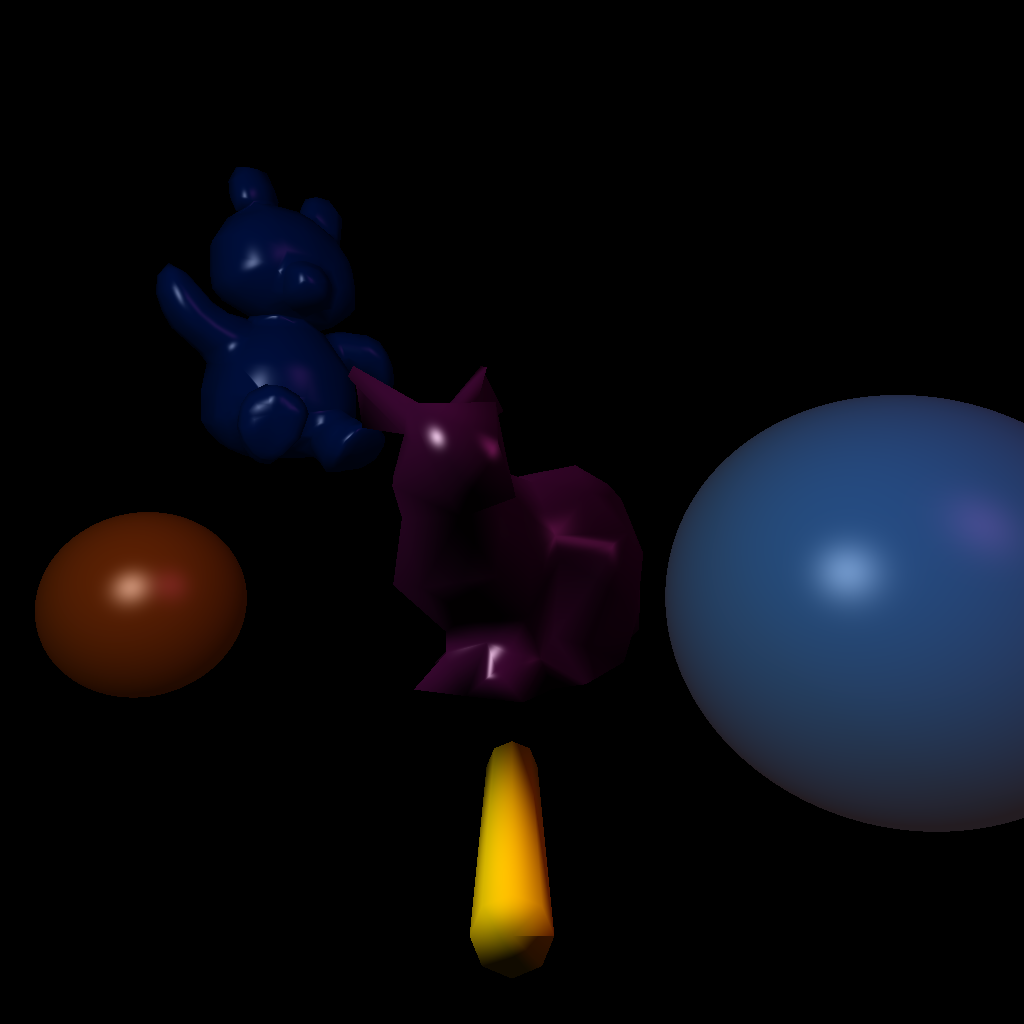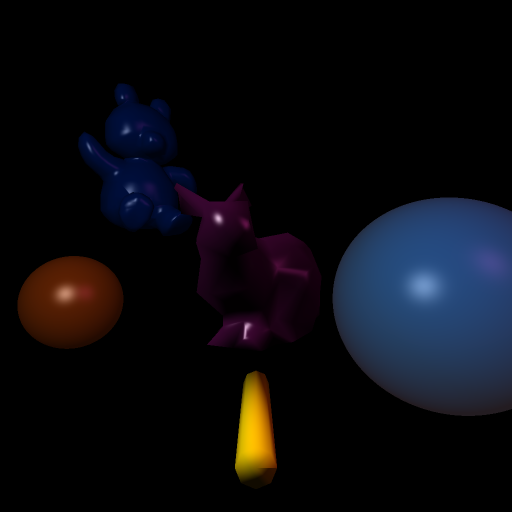# CS 636 Programming Assignment 2 - Add diffuse/specular shading model with point lights

## First Scene Setup

The camera for the scene is setup at (0,0,4) for the eye point, with a view direction of (0,0,-1) and a view up direction of (.3,1.0), normalized, to tilt the scene so it is not all right angles. The view plane distance is 6, and the horizontal camera angle is PI / 6.

The scene has two point light sources: a white light at (-5, 5, 5), and light source with color (0.4, 0.1, 0.3) rgb at position (-3, 20, 5).

The scene contains 5 objects: two spheres and three mesh objects including a flat shaded cube and a smooth shaded cylinder and finally a smooth shaded bunny.

The scene took 433 seconds to render on my PC laptop. Rerendering it on my Mac Mini M1 took only 15 seconds!.

### Bunny

The bunny is translated along the z axis +2 to move it closer to the camera from the original mesh in "bound-bunny_200.smf". It is also rotated 45 degrees around the Y axis.
• Diffuse surface color of purple (r,g,b) = (0.7, 0.1, 0.6)
• Specular surface color of white (r,g,b) = (1,1,1)
• Ambient surface color of purple (r,g,b) = (0.7, 0.1, 0.6)
• Diffuse coefficient of 0.8.
• Specular coefficient of 0.5
• Ambient coefficient of 0.1
• Specular exponent of 2

### First Sphere

The first sphere (on the right) is centered at (8, -2, -10) with a radius of 4.5.
• Diffuse surface color of medium blue (r,g,b) = (3/255, 83/255, 151/255)
• Specular surface color of white (r,g,b) = (1,1,1)
• Ambient surface color of white (r,g,b) = (1,1,1)
• Diffuse coefficient of 0.8.
• Specular coefficient of 0.4
• Ambient coefficient of 0.1
• Specular exponent of 5

### Second Sphere

The second sphere (on the left) is centered at (-8, -2, -10) with a radius of 4.5.
• Surface color(diffuse,specular, and ambient) orange (r,g,b) = (232/255, 99/255, 10/255)
• Diffuse coefficient of 0.8.
• Specular coefficient of 0.1
• Ambient coefficient of 0.1
• Specular exponent of 1.5

### Cube

The cube mesh object is translated from the original SMF model to translate it up (2.0) along the y axis. The cube is rendered with flat shading(without interplating normals).
• Surface color(diffuse,specular, and ambient) dark blue (r,g,b) = (0, 30/255, 108/255)
• Diffuse coefficient of 0.8.
• Specular coefficient of 0.1
• Ambient coefficient of 0.1
• Specular exponent of 2

### Cylinder

The cylinder mesh object is translated from the original SMF model to translate it down (-2.0) along the y axis.
• Surface color(diffuse,specular, and ambient) of yellow (r,g,b) = (252/255, 217/255, 0)
• Diffuse coefficient of 0.8.
• Specular coefficient of 0.05
• Ambient coefficient of 0.05
• Specular exponent of 2

## Original 1024 by 1024 Image## Smoothed 512 by 512 Image## Second Scene Setup

I added this scene to show better examples of specular highlights.

The camera for the scene is setup at (0,0,4) for the eye point, with a view direction of (0,0,-1) and a view up direction of (0, 1,0 ). The view plane distance is 6, and the horizontal camera angle is PI / 6.

The scene has two point light sources: a white light at (-5, 5, 5), and light source with color (0.4, 0.1, 0.3) rgb at position (13, 10, 5);

The scene contains 5 objects: two spheres and three mesh objects including a and a smooth shaded cylinder, a smooth shaded bunny, and a smooth shaded teddy bear.

Rendering it on my Mac Mini M1 took 192 seconds (much longer with teddy instead of cube from first scene).

### Bunny

The bunny is translated along the z axis +2 to move it closer to the camera from the original mesh in "bound-bunny_200.smf". It is also rotated 45 degrees around the Y axis.
• Diffuse surface color of purple (r,g,b) = (0.7, 0.1, 0.6)
• Specular surface color of white (r,g,b) = (1,1,1)
• Ambient surface color of purple (r,g,b) = (0.7, 0.1, 0.6)
• Diffuse coefficient of 0.3
• Specular coefficient of 0.7
• Ambient coefficient of 0.01
• Specular exponent of 30

### First Sphere

The first sphere (on the right) is centered at (8, -2, -10) with a radius of 4.5.
• Diffuse surface color of medium blue (r,g,b) = (3/255, 83/255, 151/255)
• Specular surface color of white (r,g,b) = (1,1,1)
• Ambient surface color of white (r,g,b) = (1,1,1)
• Diffuse coefficient of 0.8.
• Specular coefficient of 0.4
• Ambient coefficient of 0.1
• Specular exponent of 25

### Second Sphere

The second sphere (on the left) is centered at (-8, -2, -10) with a radius of 2.
• Surface color(diffuse and ambient) of orange (r,g,b) = (232/255, 99/255, 10/255)
• Specular surface color of white (r,g,b) = (1,1,1)
• Diffuse coefficient of 0.3.
• Specular coefficient of 0.6
• Ambient coefficient of 0.1
• Specular exponent of 15

### Cylinder

The cylinder mesh object is translated from the original SMF model to translate it down (-2.0) along the y axis.
• Surface color(diffuse,specular, and ambient) of yellow (r,g,b) = (252/255, 217/255, 0)
• Diffuse coefficient of 0.8.
• Specular coefficient of 0.05
• Ambient coefficient of 0.05
• Specular exponent of 2

The mesh for the teddy bear is translated by (-5, 4, -10), scaled by a factor of 0.15, and rotated around Y axis by PI / 4.

### Teddy Bear

• Surface color (diffuse and ambient) dark blue (r,g,b) = (0, 30/255, 108/255)
• Specular surface color of white (r,g,b) = (1,1,1)
• Diffuse coefficient of 0.5
• Specular coefficient of 0.5
• Ambient coefficient of 0.1
• Specular exponent of 25

## Original 1024 by 1024 Image## Smoothed 512 by 512 Image